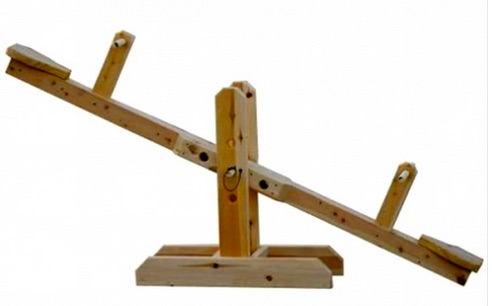# RATL 1 | Lesson 4 | Explore (Multiple Strategies Solutions)

Here are some different ways to think about the solution to the problem:

How many pounds of coffee worth $7 per pound must be mixed with 12 pounds of coffee worth$4 per pound to make a mixture worth $6 per pound? 1. Creating an Equation: Let $$x$$ represent the number of pounds of the$7 per pound coffee. There are 12 pounds of the $4 per pound coffee and $$x$$ pounds of$7 per pound mixture means that the total is $$x+12$$pounds.

Knowing the cost for each type of coffee gives us this equation to solve:

\begin{align}4(12)+7(x)&=6(x+12)\\\\48+7x&=6x+72\\\\x&=24\end{align}

, $$24$$ pounds of the $$7$$ per pound coffee is needed.

2. Creating a Table:

Number of Pounds Cost Per Pound Total Cost
Cheaper Coffee $$12$$ $$4$$ $$48$$
Expensive Coffee $$x$$ $$7$$ $$7x$$
Combined Coffee $$12+x$$ $$6$$ $$6(12+x)$$

Use the Total Cost Column to create this equation to solve:

\begin{align}48+7(x)&=69x+12)\\\\48+7x&=6x+72\\\\x&=24\end{align}

Notice the solution is $$24$$.

3. Using a Balance :http://www.gardenista.com/products/teeter-totter

$$12$$               $$12+x$$                $$x$$         weight

________________________

$$4$$                $$6$$                  $$7$$       cost per lb

Set up a proportion using the weight in pounds and difference between price per pound.

$(6–4 =2) \text{ and } (7–6 = 1)$

$\dfrac{12}{1}=\dfrac{x}{2}$

Solve the proportion:

$$x=24$$